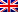#[Internetwache CTF 2016] [Exploit 60 – EquationSolver] Write Up

## Description

I created a program for an unsolveable equation system. My friend somehow forced it to solve the equations. Can you tell me how he did it?

Service: 188.166.133.53:12049

## Resolution

We connect to the service, and discover what is this unsolveable equation.

```vic511@vic511:~/ctfs/internetwache2k16\$ nc 188.166.133.53 12049
Solve the following equations:
X > 1337
X * 7 + 4 = 1337
Enter the solution X:
```

The first thing we think about is integer overflow, the following test admits the remote binary is 32 bits.

```vic511@vic511:~/ctfs/internetwache2k16\$ python -c 'print 2**30' | nc 188.166.133.53 12049
Solve the following equations:
X > 1337
X * 7 + 4 = 1337
Enter the solution X: You entered: 1073741824
1073741824 is bigger than 1337
-1073741820 is not equal to 1337
WRONG!!!
Go to school and learn some math!
```

Nice ! Our hypothesis was right.
The equation should be solved this way:

```X * 7 + 4 = 1337
⟺ X = (1337 - 4) / 7
⟺ X = int(190.4285) = 190
```

This solution is obviously under 1337, so it is rejected by the program. But what if we consider X as a 33 bit integer, and set the most significant bit to 1 ? It should still be interpreted as 1337 as a 32 bit integer, isn’t it ?
Let’s use the following python script to predict the result of the calculus done by the binary.

```num = ((2**32 + 1337) - 4) / 7; # the number we're using to "solve" the equation
num = (num * 7 + 4) & (2**32 - 1); # how it will be computed to verify the equality as a 32 bit integer
print ((num) & (2**31 - 1)) * (-1 if num >> 31 else 1) # bitwise tricks to foresee the real value
```

This outputs 1337 ! Let’s see if this is what actually happens service-side.

```vic511@vic511:~/ctfs/internetwache2k16\$ python -c 'print (2**32+1337-4)/7' | nc 188.166.133.53 12049
Solve the following equations:
X > 1337
X * 7 + 4 = 1337
Enter the solution X: You entered: 613566947
613566947 is bigger than 1337
1337 is equal to 1337
Well done!
IW{Y4Y_0verfl0w}
```

Great, we have the flag !
`IW{Y4Y_0verfl0w}`